# Understanding Forces on Current-Carrying Wires in Magnetic Fields

Lesson Transcript
Instructor: Betsy Chesnutt

Betsy has a Ph.D. in biomedical engineering from the University of Memphis, M.S. from the University of Virginia, and B.S. from Mississippi State University. She has over 10 years of experience developing STEM curriculum and teaching physics, engineering, and biology. She currently teaches first year engineering students at the University of Tennessee.

Magnetic fields exert forces on current-carrying wires. In this lesson, learn how magnetic fields are created and calculate the force a magnetic field will exert on a current-carrying wire.

## Magnetic Fields

What makes something magnetic? Although people have used naturally occurring magnets for thousands of years, we didn't understand how to create a magnetic field until about 200 years ago.

In 1819, a Danish scientist and teacher named Hans Christian Oersted was demonstrating how to create a current in a wire by connecting it to a battery when he happened to notice that a magnetic compass lying near the wire would turn every time the wire was connected to the battery. He quickly realized that the current in the wire was creating a magnetic field that was affecting the compass.

If a current-carrying wire can create a magnetic field, do you think that a magnetic field also affects a current-carrying wire? Of course! When any current-carrying wire is placed in a magnetic field, the magnetic field exerts a force on the wire. Magnetic field strength is commonly measured in units of Tesla, which is abbreviated T.

1 T = 1 kg/s2 *A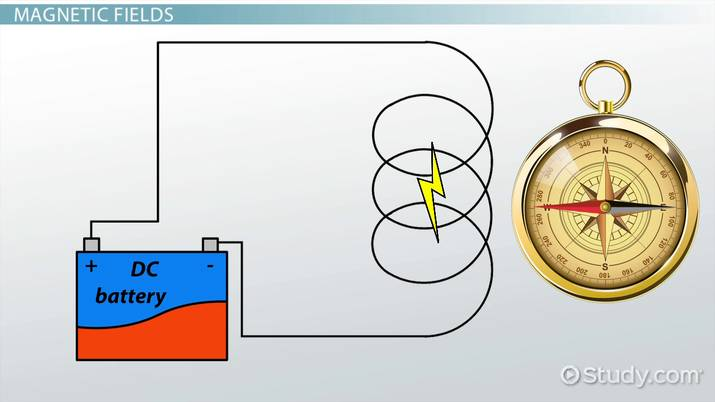An error occurred trying to load this video.

Try refreshing the page, or contact customer support.

Coming up next: Electromagnetic Induction: Definition & Variables that Affect Induction

### You're on a roll. Keep up the good work!

Replay
Your next lesson will play in 10 seconds
• 0:04 Magnetic Fields
• 1:00 Straight Current-Carrying Wire
• 3:18 Two Parallel…
• 4:47 Current Loops
• 5:15 Lesson Summary
Save Save

Want to watch this again later?

Timeline
Autoplay
Autoplay
Speed Speed

## Straight Current-Carrying Wire

When a long, straight current-carrying wire is placed in a magnetic field, it'll experience a force proportional to the strength of the field, the amount of current, and the length of the wire. You can see how this plays out in this image: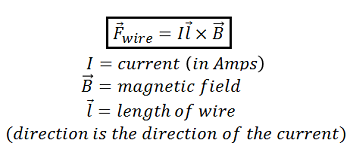Thus, the magnitude of the magnetic force on a current-carrying wire is given by: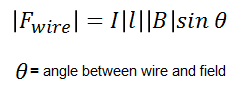The direction of the magnetic field can be found using the right-hand rule. To determine the direction, hold up your right hand (the left won't work!). Point your index finger in the direction of the current and your middle finger in the direction of the field. Your thumb will point in the direction of the magnetic force.

Let's look at an example. The wire shown is being levitated by a magnetic field. What is the magnitude and direction of the magnetic field necessary to levitate this wire?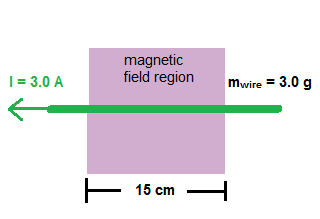First, determine what magnetic force must be exerted on the wire. If the wire is suspended in the air, there are two forces acting on it. There is the downward force of gravity and an upward force due to the magnetic field. Therefore, the magnetic force acting upward on the wire must be equal to the force of gravity acting downward.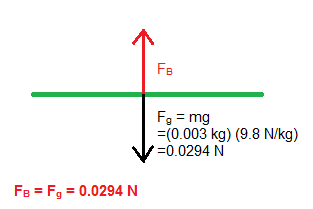Now, you can calculate the magnitude of the magnetic field required to exert this force, making sure to use the formula we explored earlier. To get the maximum force with the smallest magnetic field, the field should be perpendicular to the current direction.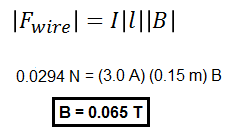Finally, use the right-hand rule to find the direction of the magnetic field. Use your right hand and point your index finger in the direction of the current (left) and your thumb in the direction of the force (up). Then, your middle finger will point in the direction that the field should be directed.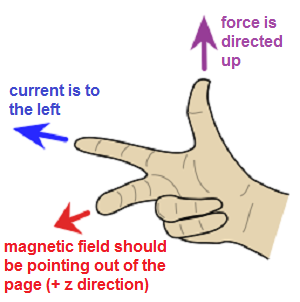Finally, use the right-hand rule to find the direction of the magnetic field. Use your right hand and point your index finger in the direction of the current (left) and your thumb in the direction of the force (up), then your middle finger will point in the direction that the field should be directed. So, to levitate this current-carrying wire, you would need a 0.065 T magnetic field directed outward in the +z direction.

To unlock this lesson you must be a Study.com Member.

### Register to view this lesson

Are you a student or a teacher?